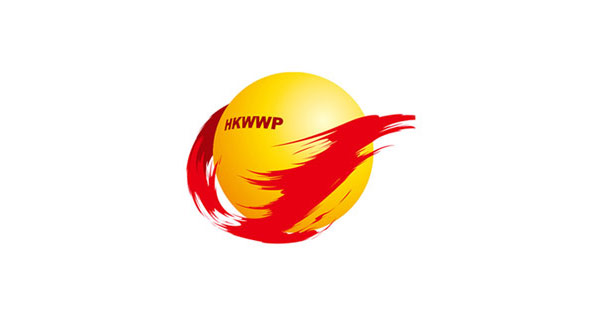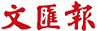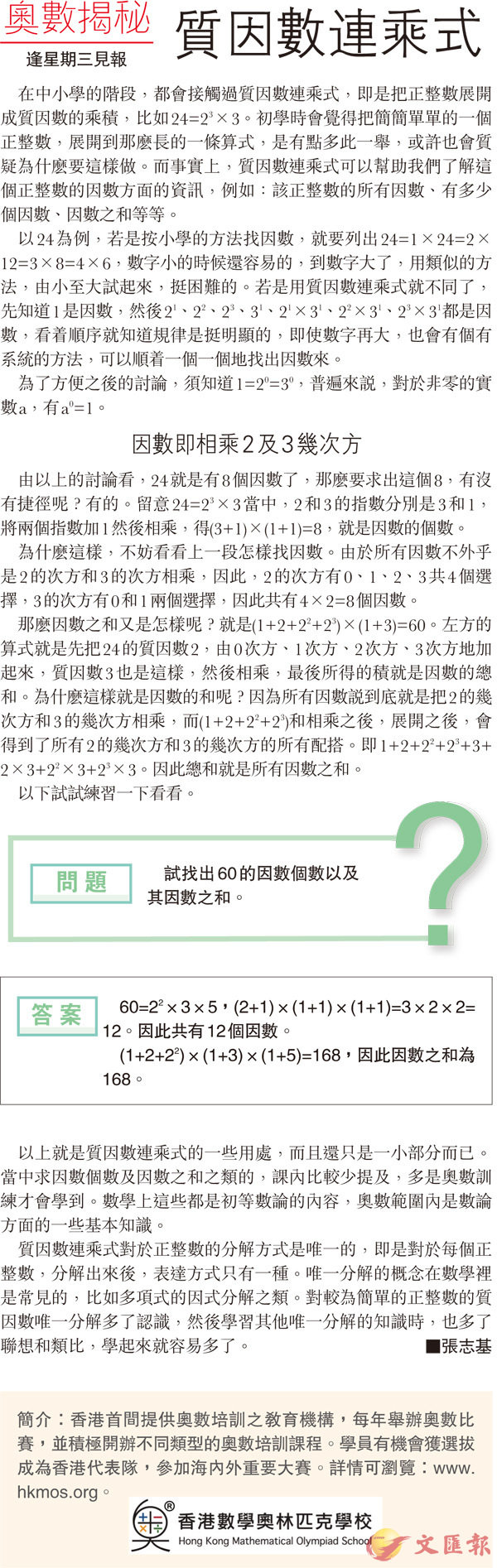首頁 > 文匯報 > 百科啟智 > 正文

# 【奧數揭秘】質因數連乘式

2017-11-2960=22×3×5，(2+1)×(1+1)×(1+1)=3×2×2=12。因此共有12個因數。

(1+2+22)×(1+3)×(1+5)=168，因此因數之和為168。